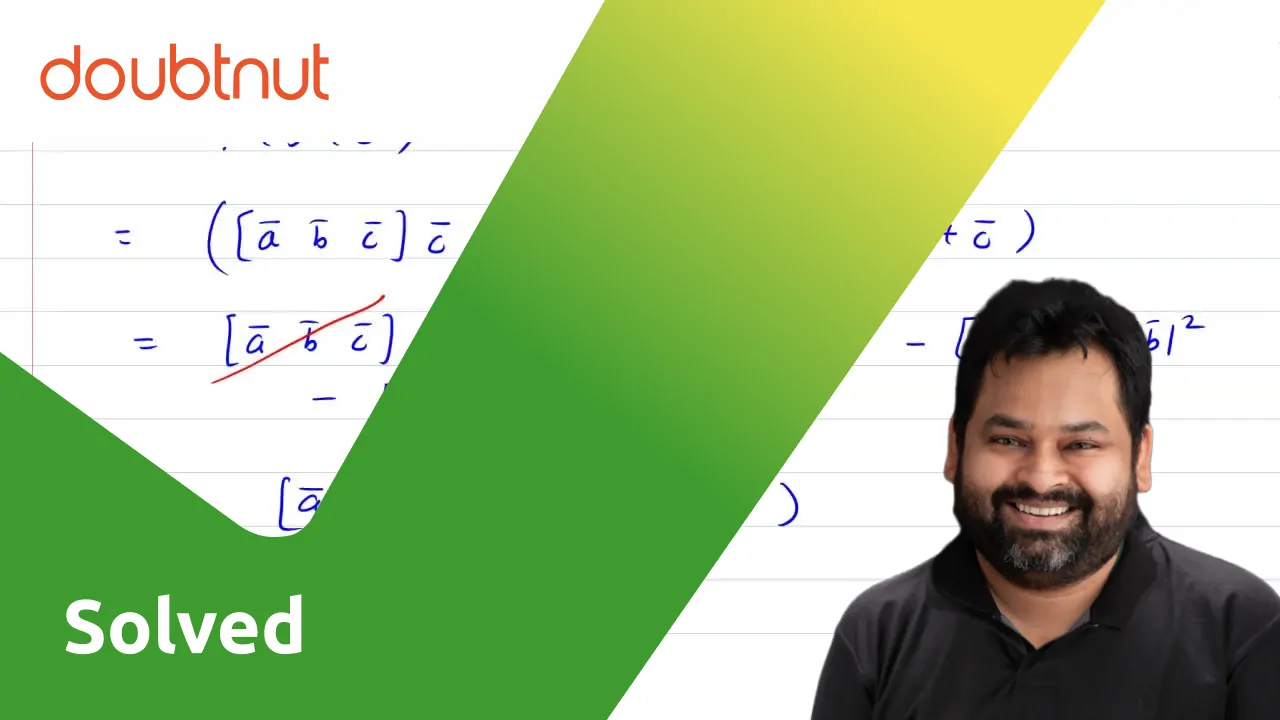Doubtnut App - Get 50 Lakh+ video solutions better than GoogleInstall Now

# If →a,→b,→c are vectors such that ∣∣→b∣∣=|→c| then {(→a+→b)×(→a+→c)}×(→b×→c).(→b+→c)=

Text Solution
Verified by Experts

## We have (→A+→B)×(→A+→C) =→A×→A+→B×→A+→A×→C+→B×→C =→B×→A+→A×→C+→B×→C (→A×→A=→0) [(→A+→B)×(→A+→C)]×(→B×→C) [→B×→A+→A×→C+→B×→C]×(→B×→C) {(→B×→A).→C)→B−{(→B×→A).→B}→C +{(→A×→C).→C}→B−{(→B−→C).(→B+→C)} [→B→A→C]→B−[→A→C→B]→C [→A→C→B]{→B−→C} thus, L.H.S of the given expression becomes [→A→C→B](→B−→C).(→B+→C) [→A→C→B]{∣∣→B∣∣2−∣∣→C∣∣2}= (∣∣→B∣∣=∣∣→C∣∣) Altenate method Since [(→A+→B)×(→A+→C)]×(→B+→C).(→B+→C) is a scalar triple product of (→A+→B)×(→A+→C),→B+→Cand→B+→C its value is 0.